# Java String split: How to Split String in Java

While programming, we may need to break the String based on some attributes. Mostly this attribute will be a separator or a common – with which you want to break or split the String.

## Java String split()

Java String split() is a built-in function that splits the String wherever it encounters a given regex and returns the split up parts in the form of an array. The string split() method breaks a given string around matches of the given regular expression. In this example, you will learn how to split the String in Java.

To convert Java string to array, use the string split() function.

Java String split() method has the following two variations:

1. public String [] split(String regex, int limit)
2. public String [] split(String regex)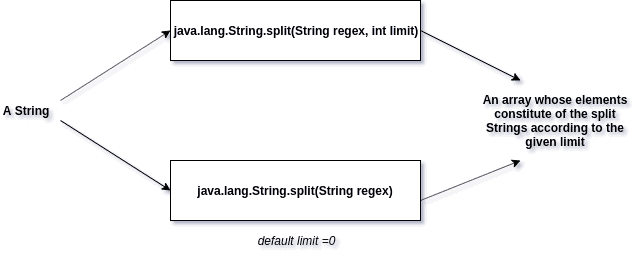## Internal Implementation

Let’s see an internal implementation of the Java String split() function.

```public String[] split(String regex, int limit) {
/* fastpath if the regex is a
(1)one-char String and this character is not one of the
RegEx's meta characters ".\$|()[{^?*+\\", or
(2)two-char String and the first char is the backslash and
the second is not the ascii digit or ascii letter.
*/
char ch = 0;
if (((regex.value.length == 1 &&
".\$|()[{^?*+\\".indexOf(ch = regex.charAt(0)) == -1) ||
(regex.length() == 2 &&
regex.charAt(0) == '\\' &&
(((ch = regex.charAt(1))-'0')|('9'-ch)) < 0 &&
((ch-'a')|('z'-ch)) < 0 &&
((ch-'A')|('Z'-ch)) < 0)) &&
(ch < Character.MIN_HIGH_SURROGATE ||
ch > Character.MAX_LOW_SURROGATE))
{
int off = 0;
int next = 0;
boolean limited = limit > 0;
ArrayList<String> list = new ArrayList<>();
while ((next = indexOf(ch, off)) != -1) {
if (!limited || list.size() < limit - 1) {
off = next + 1;
} else {    // last one
//assert (list.size() == limit - 1);
off = value.length;
break;
}
}
// If no match was found, return this
if (off == 0)
return new String[]{this};

if (!limited || list.size() < limit)

// Construct result
int resultSize = list.size();
if (limit == 0)
while (resultSize > 0 && list.get(resultSize - 1).length() == 0)
resultSize--;
String[] result = new String[resultSize];
return list.subList(0, resultSize).toArray(result);
}
return Pattern.compile(regex).split(this, limit);
}
```

## Passing a limit as a parameter

### Syntax

```public String [] split(String regex, int limit)
```

### Parameters:

String regex: The regular expression encountering which the split should occur.

int limit: An integer that specifies the maximum number of splits depending on whether it is positive, negative, or zero.

Returns: An array of Strings whose items are constituted by splitting the initial String accordingly.

Throws:  On invalid regex syntax, throws PatternSyntaxException.

The first variation of the split() method requires that the programmer passes a limit parameter, which specifies the number of times the String in question is supposed to be split.

If the limit is positive, then the String is to be split amongst limit-1 times (on encountering the given regex), and the resulting array can then contain almost a limit number of elements.

Let’s see the following code.

```public class Example1 {
public static void main(String[] args) {
String s1 = new String("This-is-a-split-example--");
String[] arr = s1.split("-", 5);

for (String x : arr)
System.out.println(x);
}
}```

#### Output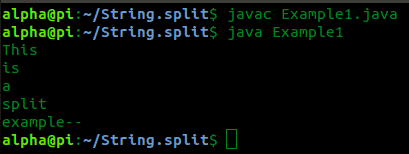In the above example, we have split the String from the – (hyphen) symbol.

Let’s see another example.

```public class Example2 {
public static void main(String[] args) {
String s1 = new String("This-is-a-split-example--");
String[] arr = s1.split("-", 6);

for (String x : arr)
System.out.println(x);

}
}
```

#### Output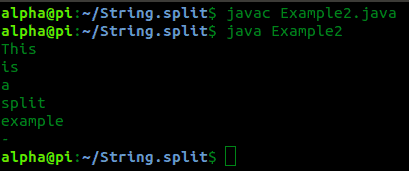See another example.

```public class Example3 {
public static void main(String[] args) {
String s1 = new String("This-is-a-split-example--");
String[] arr = s1.split("-", 7);

for (String x : arr)
System.out.println(x);
}
}
```

#### Output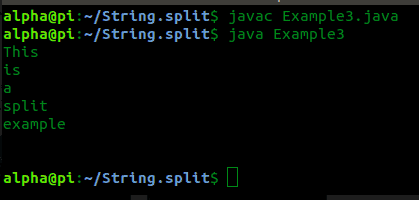If the limit is negative, then the String can be split any number of times (on encountering the given regex), and the resulting array can then contain any number of elements.

If there is nothing adjacent to the regex for splitting, then a space is added to the array in such a case.

See the following code example.

```public class Example4 {
public static void main(String[] args) {
String s1 = new String("This-is-a-split-example--");
String[] arr = s1.split("-", -2);

for (String x : arr)
System.out.println(x);

}
}
```

#### Output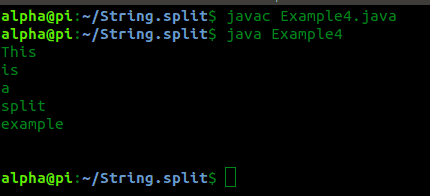If the limit is zero, the String can be split any number of times(on encountering the given regex). The resulting array can then contain any number of elements, as in the case of a negative limit. But the trailing empty spaces are not included in the array in this case.

See another example.

```public class Example5 {
public static void main(String[] args) {
String s1 = new String("This-is-a-split-example--");
String[] arr = s1.split("-", 0);

for (String x : arr)
System.out.println(x);
}
}
```

#### Output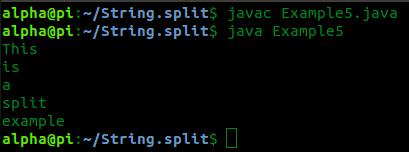## Without passing a limit as a parameter

Syntax: public String [] split(String regex)

Parameters:  String regex: The regular expression encountering the split should occur.

Returns: An array of Strings whose i are constituted by splitting the initial String accordingly. Here, the limit is set to zero by default, and hence, the resulting array is generated.

Throws:  On invalid regex syntax, throws PatternSyntaxException.

See the string split() example with regex.

```public class Example6 {
public static void main(String[] args) {
String s1 = new String("This-is-a-split-example--");
String[] arr = s1.split("-");

for (String x: arr)
System.out.println(x);

}
}```

#### Output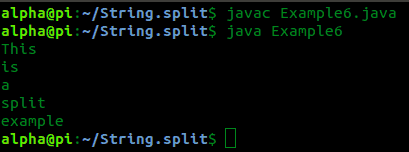That’s it for this tutorial.

## Related Posts

Java String join()

Java String intern()

Java String replace()

Java String replaceAll()

Java String format()

This site uses Akismet to reduce spam. Learn how your comment data is processed.